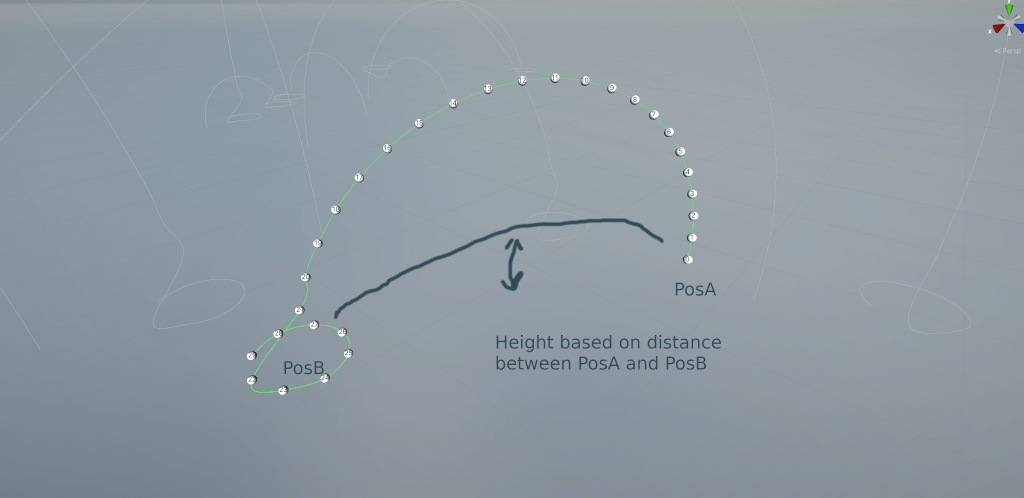# Control height of arc of points between gameobjects

I have a functioning script that creates an arc of waypoints for a cinemachine track between 2 gameobject(vector3's), I'd like to add a variable so that I can control the height of the arc as seen in this pic:At the moment the method I have creates the arc based on a radius of the distance between the 2 points, I'd like to be able to control the height of the arc so that it calculates the points similar to the drawn line in the pic. And to control where in the arc that maximum height occurs in relationship to the distance between posA and posB.

Here's the code I have for creating the arc as it is at the moment, which calculates the arc with a radius of half the distance between the 2 points:

``````static void CalcWaypoints(CinemachineSmoothPath path, Vector3 posA, Vector3 posB)
{
int greenRingPointsNum = 8;
float metersPerWaypoint = 10;
//Here we calculate how many segments will fit between the two points
int segmentsToCreate = Mathf.RoundToInt(Vector3.Distance(posA, posB) / metersPerWaypoint);
path.m_Waypoints = new CinemachineSmoothPath.Waypoint[segmentsToCreate + greenRingPointsNum];
Debug.Log("Creating " + segmentsToCreate + " waypoints");

// get circle center and radius
var radius = Vector3.Distance(posA, posB) / 2f;
var centerPos = (posA + posB) / 2f;

// get a rotation that looks in the direction of the target gameobject
var centerDirection = Quaternion.LookRotation((posA - posB).normalized);

for (var i = 0; i < segmentsToCreate; i++)
{

var angle = Mathf.PI * (i) / (segmentsToCreate + 1f);
var y = Mathf.Sin(angle) * radius;
var z = Mathf.Cos(angle) * radius;
var pos = new Vector3(0, y, z);
// Rotate the pos vector according to the centerDirection
pos = centerDirection * pos;
path.m_Waypoints[i] = new CinemachineSmoothPath.Waypoint();
path.m_Waypoints[i].position = centerPos + pos;
}

//create a circle of points around the target gameobject at a give radius
int waypointNum = segmentsToCreate;
for (int i = 0; i < greenRingPointsNum; i++)
{
float angle = i * Mathf.PI * 2f / greenRingPointsNum;
Vector3 newPos = posB + new Vector3(Mathf.Cos(angle) * greenRadius, posB.y, Mathf.Sin(angle) * greenRadius);
path.m_Waypoints[waypointNum] = new CinemachineSmoothPath.Waypoint();
path.m_Waypoints[waypointNum].position = newPos;
waypointNum++;
}

}
``````

Hope one of you great folks can help out :)

An Arc like the following pic, where I can control the height and it's distance between the 2 points: